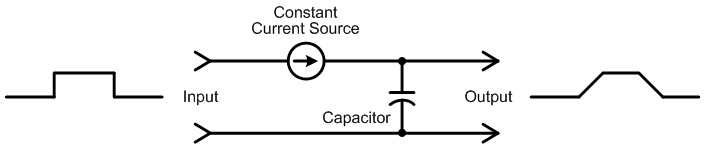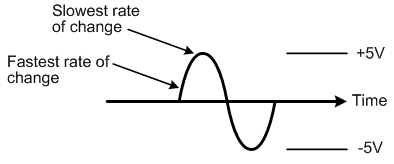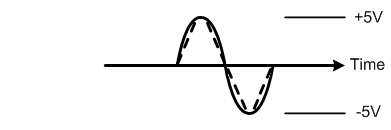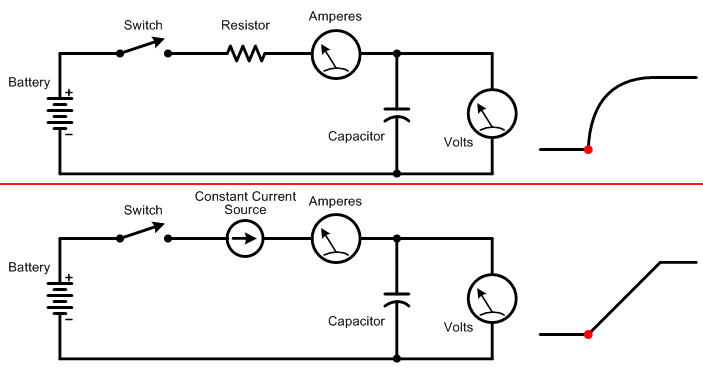www.ethanwiner.com - since 1997
 Home & Contact Audio & Music Bio Programming Wisdoms & Quotes Funny Names Magazine Articles Cello Page Ethan's Tunes Oxymorons Family Photos

Slew Rate and Slew Rate Limiting

This article complements the section that describes slew rate on page 595 of my book The Audio Expert (first edition only).

The terms slew rate and slew rate limiting are often used to describe the performance of audio devices, but many people don't fully understand what these terms mean. The formal definition of slew rate is how quickly a circuit's output voltage can change, and it's usually expressed in volts per microsecond. This is different from frequency response, which is independent of output voltage. If a power amplifier is 3 dB down at 50 KHz, it will be reduced by that much whether the input signal is 0.1 volts or 10 volts. However, a circuit's maximum output capability at high frequencies is a function of its high frequency response and also its slew rate. A preamp might be flat to 50 KHz when passing small signals, but unable to output even 10 KHz at very high levels without distortion.

In order to learn how slew rate differs from regular frequency response, we first need to understand how capacitors charge and discharge over time. A typical low-pass filter circuit uses resistors and capacitors to limit how quickly the output can track the input. In the filter shown in Figure 1 below, a resistor limits the amount of current available to charge the capacitor. It takes time for a capacitor to charge or discharge, not unlike a rechargeable battery. So rapid voltage changes at the input - either rising or falling - do not make it through to the output. Eventually when the frequency is high enough, nothing at all appears at the output.Figure 1: Sending audio through a series resistor and shunt capacitor as shown creates a low-pass filter. Rapid changes at the input do not pass through to the output because it takes time for the capacitor to charge and discharge.

All audio circuits contain some amount of "stray" capacitance due to the close proximity of connecting wires, PC board traces, and electronic components. Indeed, a capacitor is made from two or more plates of metal that are very close together, but not quite touching. So even when a circuit is meant only to amplify, and not alter the frequency response like a filter, stray capacitance limits the response at very high frequencies. Many amplifiers also include "compensation" capacitors that intentionally roll off very high frequencies above the audible range to prevent ultrasonic oscillation.

You may wonder why charging a capacitor through a resistor creates an output voltage that rises as a curve rather than as a straight line. This is because less and less current is drawn by the capacitor as it charges. When the input voltage first jumps up from zero volts, the capacitor acts as a short circuit across the top and bottom signal wires. So the full input voltage is sent through the resistor, which in turn draws some amount of current. As the capacitor charges, less voltage goes through the resistor, which in turn causes less current to be drawn. For example, when the capacitor is halfway charged, half of the input voltage is across the resistor and the other half is across the capacitor. So only half as much current is drawn through the resistor. When the capacitor is almost fully charged, only a very small amount of current passes through the resistor, further slowing the charging rate.

The charging rate of a capacitor is the same for small or large signals. In other words, the output reaches 80 percent of the input voltage after the same amount of time whether the input is 0.1 volt or 10 volts. However, when the input voltage becomes large enough, the amplifier's transistors or op-amps may be unable to supply enough current to charge the stray capacitance fast enough to pass high frequencies. At that point the circuit goes into current-limiting, which limits how quickly the stray capacitance can charge. Figure 2 below shows the same stepped input signal as Figure 1, but with the resistor replaced by a current source that outputs a constant amount of current regardless of the capacitor's present charge level.Figure 2: Charging (or discharging) a capacitor from a constant current source causes the output voltage to rise (or fall) at a constant rate.

This difference in charging rates using a resistor versus a current source is significant because a sine wave's rate of change varies at different points along its cycle. As you can see in Figure 3 below, the initial portion of a sine wave rises rapidly compared to the flatter portion along the top.Figure 3: A sine wave's voltage rises and falls faster at some parts of its cycle than at others.

When the amount of current available to charge the circuit's stray capacitance is limited, the fast rising slope of the sine wave becomes more like a triangle wave as shown in Figure 4 below. This change in wave shape creates distortion.Figure 4: When insufficient current is available to accommodate the steep rising and falling slopes of a high frequency sine wave, it turns into a triangle wave adding new distortion frequencies.

The animation below shows the relation between input voltage, output voltage, and the amount of current drawn when charging a capacitor through a resistor, versus through a constant current source.Top: When charging a capacitor through a resistor, the initial current drawn is high, so the output voltage rises rapidly. As the capacitor charges, less and less current is drawn, which slows the rate for further charging.

Bottom: Charging a capacitor with a constant current source creates a linear rise in output voltage rather than a curve, because the charging rate is constant. If you watch both voltmeters at the same time, you'll see the upper meter rise more quickly initially, but less quickly near the end of the cycle.

This next section showing how to calculate slew rate is included only for completeness: If a preamplifier has a slew rate of 1 volt per microsecond, then it can output about 10 volts peak-to-peak at 20 KHz without adding slew rate-induced distortion. The formula to determine the minimum slew rate needed for a given frequency and output voltage is:

Slew Rate (in volts per second) = 2 * Pi * Frequency * Required Peak Output Volts

So to output 10 volts peak-to-peak at 20 KHz you need a slew rate of:

2 * 3.14 * 20,000 * 10 = 1,256,000 volts per second

Then divide the result by 1,000,000 to get microseconds:

= 1.256 volts per microsecond

Ethan Winer has been an audio pro and skeptic for most of his adult life. He now heads up RealTraps, where he designs acoustic treatment products for recording studios and home listening rooms.